Amplitude Modulation Envelope
 Home

Amplitude Modulation envelope

 -- Amplitude Modulation envelope --   5 MHz carrier modulated with 400 Hz.     using Fluke 6060B signal generator. A MAX set to 6 CM in all cases.          Formula   100 * (Amax-Amin)/(Amax+Amin) = %mod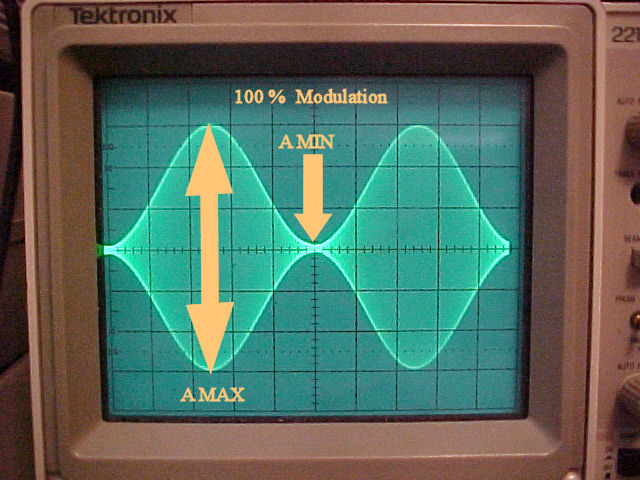A MAX = 6.00 CM A MIN = 0 CM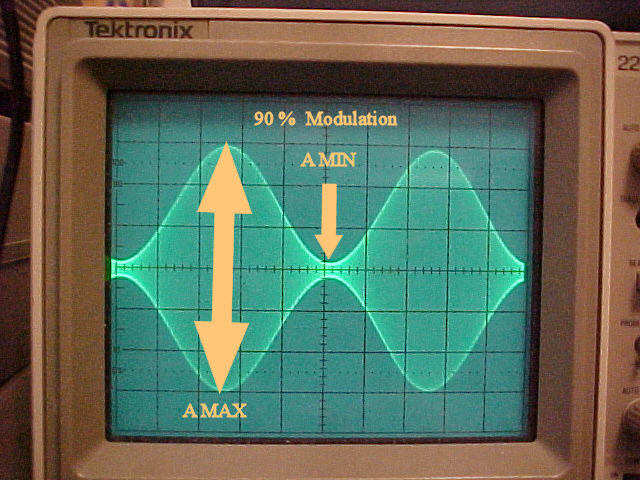A MAX = 6.00 CM A MIN = .32 CM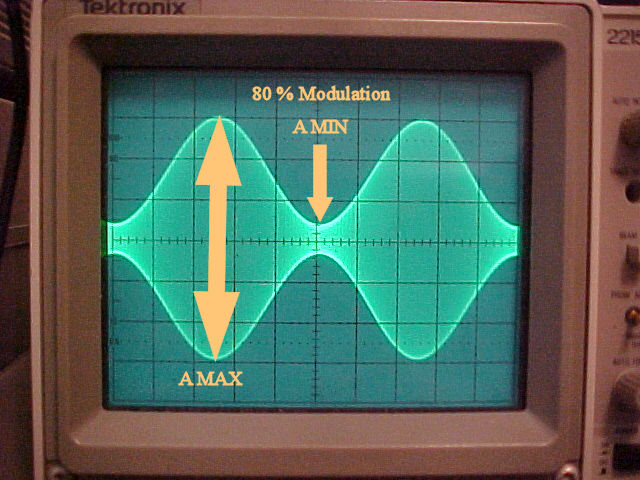A MAX = 6.00 CM A MIN = .67 CM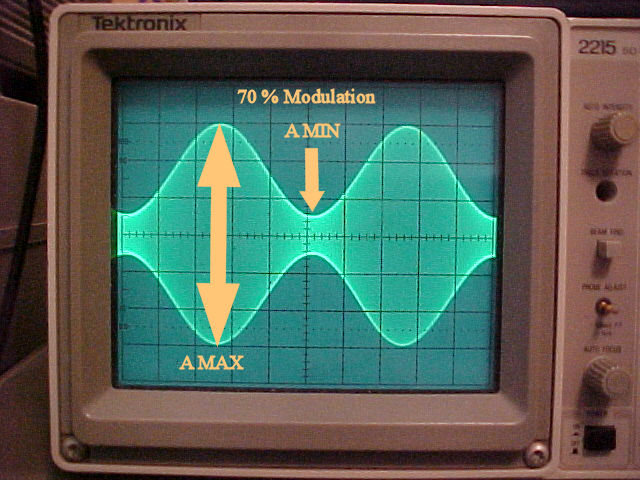A MAX = 6.00 CM A MIN = 1.06 CMA MAX = 6.00 CM A MIN = 1.50 CM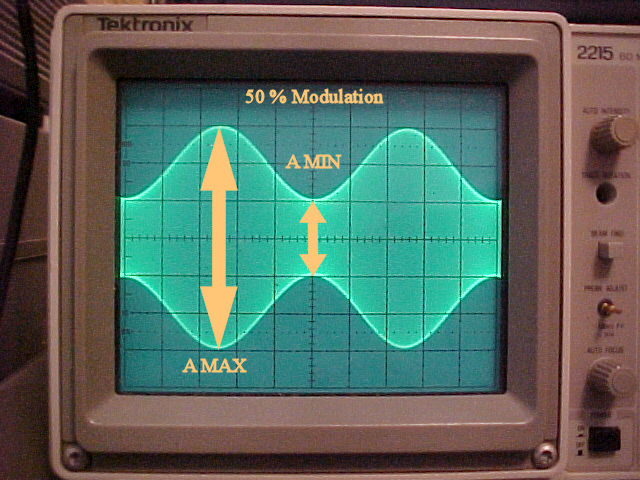A MAX = 6.00 CM A MIN = 2.00 CM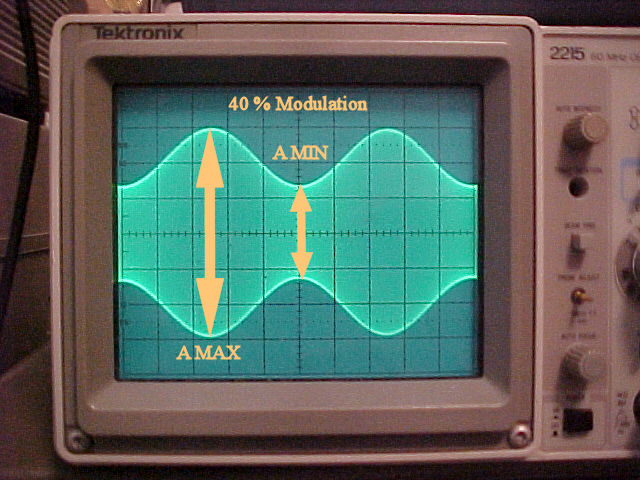A MAX = 6.00 CM A MIN = 2.57 CMA MAX = 6.00 CM A MIN = 3.23 CMA MAX = 6.00 CM A MIN = 4.00 CMA MAX = 6.00 CM A MIN = 4.91 CM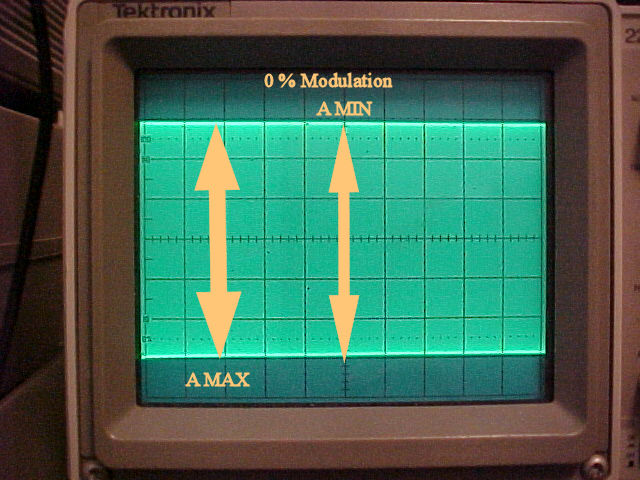A MAX = 6.00 CM A MIN = 6.00 CM